# Maths Brain Teasers 92 :: Can You Find - What Should Replace The Question Mark?

in STEMGeekslast year

Hey All; @StemGeeks Mathematician;

Without further, ado, let's get to our puzzle for today:: Maths Brain Teasers 92 :: Can You Find - What Should Replace The Question Mark?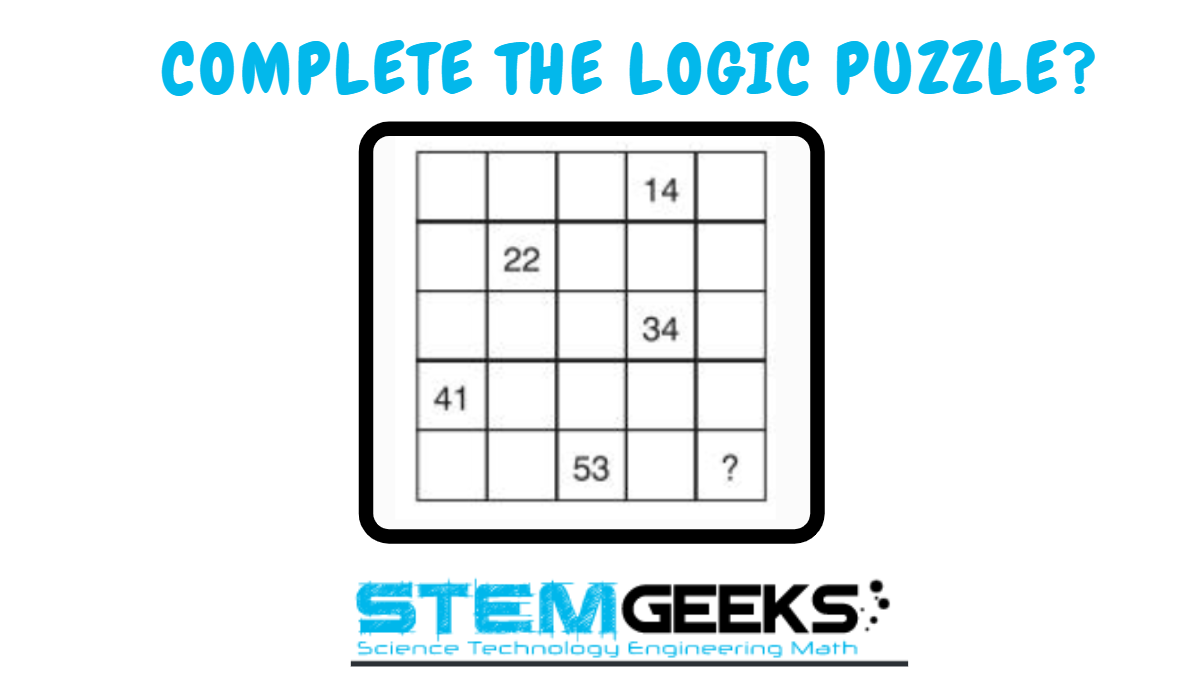Have a close look at the image given and see if you can decode the logic given in the image to complete the puzzle. Try finding out the right NUMBER that should replace the question mark. There is logic to resolve the puzzle; try finding out the logic and it should be resolved in seconds.. Guesswork isn't going to help...

With that, I'll leave you all with the Maths Brain Teasers 92 :: Can You Find - What Should Replace The Question Mark? Good luck solving the puzzle...

##### Maths Brain Teasers 91 :: Can You - Complete The Puzzle? - Solved with Explanation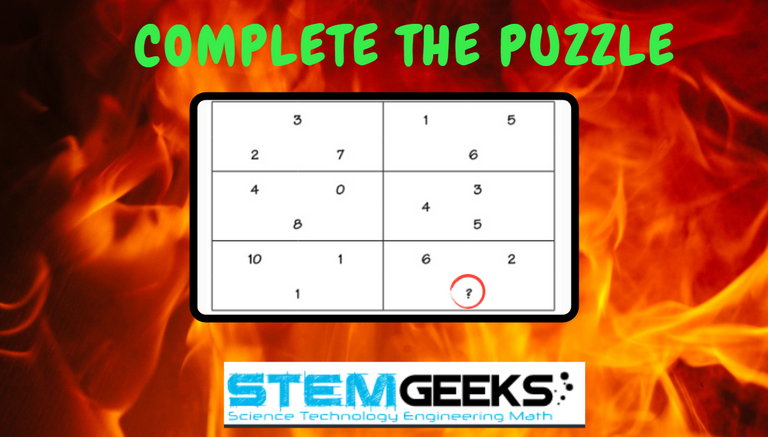Let's have a look at the Step by Step instructions to Solve the Maths Brain Teasers 91 :: Can You - Complete The Puzzle?

If you carefully look at the Image in question; You would have noticed the following pattern is being followed::

This was SUPER EASY; The Sum of all the numbers in a box is 12

Therefore the box in question is::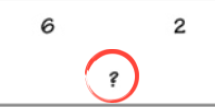### 6 + 2 + 4 = 12

The missing number is 4. The sum of three numbers in each box must be 12

### STEM token GiveAway

I'll be again doing a giveaway of STEM tokens to the lucky random winner with the correct answer. For the last contest, which was Maths Brain Teasers 91 :: Can You - Complete The Puzzle?.

We had 3 entries and I am happy to see the engagement by everyone. At the same time, it's great to see the detailed answers provided by everyone as to how they arrived at the solution of the puzzle.. Well done guys.. Way to GO... Now the results part; all the entries provided the right answer. This time, I used the random picker tool to pick one WINNER. Hence, the winner is @codingdefined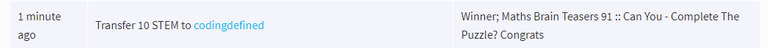Congratulations; WIN yourself 10 STEM tokens. You should be having the rewards in your STEM Wallet Soon.

### Math Quote for the Day::

Here is the motivation to solve the Maths Equation Puzzle?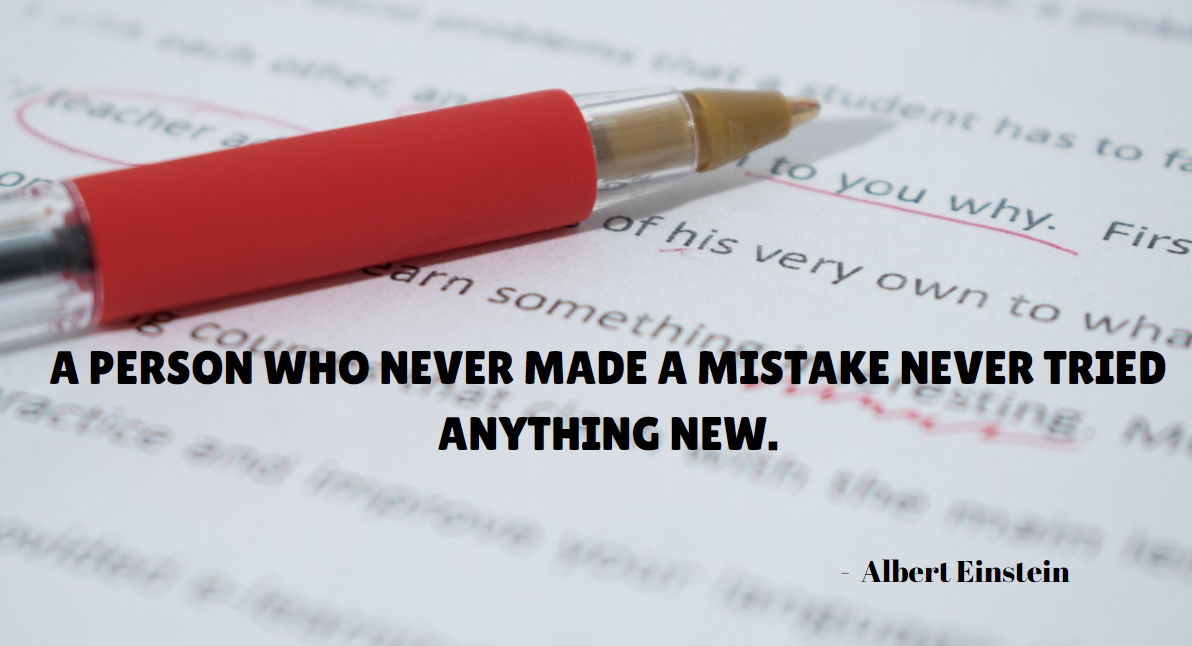If you like my work, then please spread the Word.. that we do have the Math Brain Teasers competition here @StemGeeks platform. Reblog is much appreciated.

Best RegardsFind Me on the Other Social Media Platforms::PS:: All the Maths Brain Teasers; are made by me using the Pro Canva License Version

Sort:

Confused between 71 and 59, 71 due the difference between two numbers so the last number we have to add 18 to 53, 59 by adding 6 to 53, 22-14=8, 41-34=7,59-53=6 and the other is 34-22=12, 53-41=12.

A bit tricky. I could only come up with one logic:
14+8=22
34+7=41
53+6=59

55

11+12+13+14+15/5 = 13
21+22+23+24+25/5 = 23
31+32+33+34+35/5 = 33
41+42+43+44+45/5 = 43
51+52+53+54+55/5 = 53

Well that is how I came up with 55.